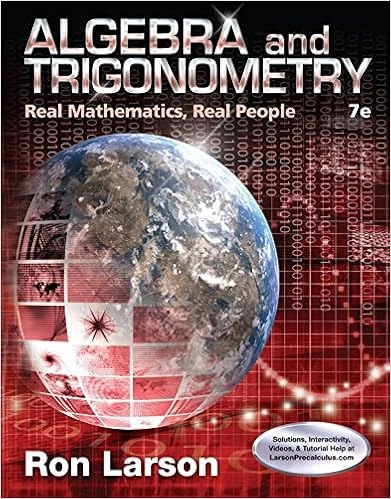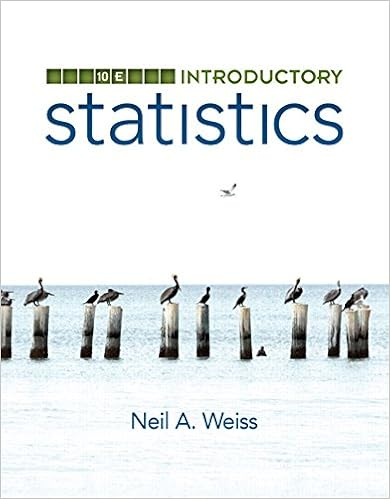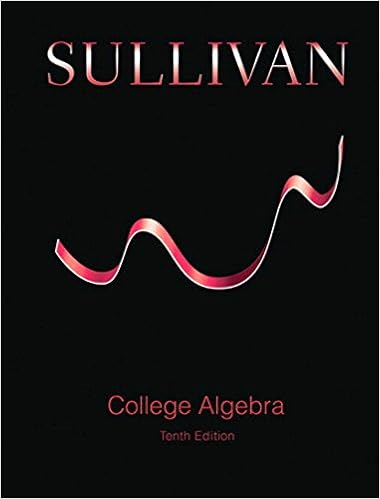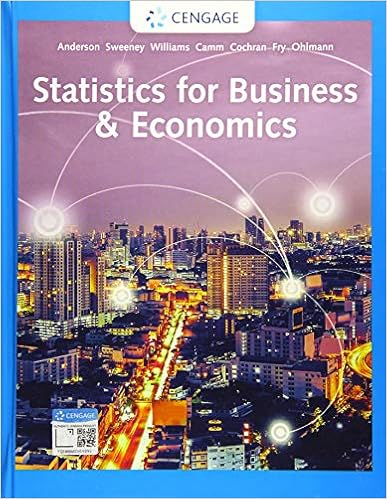# WS 1 on Definite Integrals.pdf - CALCULUS AB WORKSHEET 1 ON...

• Assignment
• 2
• 100% (1) 1 out of 1 people found this document helpful

This preview shows page 1 - 2 out of 2 pages.

CALCULUS ABNAME ______________________________________WORKSHEET 1 ON DEFINITE INTEGRALSPERIOD __________Set up a definite integral that yields the area of the region.(Do not evaluate the integral.)1.2.3.4.5.6.Sketch the region whose area is given by the definite integral.Then use a geometric formula to evaluatethe integral.7.8.9.10.11.12.Evaluate the integral using the following values:13.14.15.16.17.18.19.20.Evaluate the integral using the following values:and21.22.23.24.π/4π/2
##### We have textbook solutions for you!The document you are viewing contains questions related to this textbook.
Chapter 5 / Exercise 73
Algebra and Trigonometry: Real Mathematics, Real People
LarsonExpert VerifiedBrowse all Textbook Solutions
Evaluate the integral using the following values:and25.26.27.Evaluate the integral using the following values:and28.29.30.31.32.33. Use the table of values to estimateUse three equal subintervals and the (a) left endpoints, (b) right endpoints, and (c) midpoints.Ifan increasing function, how does each estimate compare with the actual value?is
End of preview. Want to read all 2 pages?

Course Hero member to access this document

Term
Summer
Professor
NoProfessor
Tags
following values
##### We have textbook solutions for you!
The document you are viewing contains questions related to this textbook.The document you are viewing contains questions related to this textbook.
Chapter 5 / Exercise 73
Algebra and Trigonometry: Real Mathematics, Real People
LarsonExpert Verified
•••